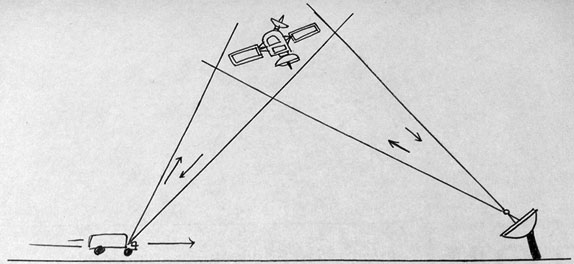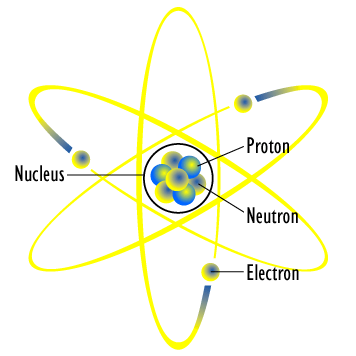## Introduction: Physics and Matter

Physics is a study of how the universe behaves.

### Learning Objectives

Apply physics to describe the function of daily life

### Key Takeaways

#### Key Points

• Physics is a natural science that involves the study of matter and its motion through space and time, along with related concepts such as energy and force.
• Matter is generally considered to be anything that has mass and volume.
• Scientific laws and theories express the general truths of nature and the body of knowledge they encompass. These laws of nature are rules that all natural processes appear to follow.

#### Key Terms

• matter: The basic structural component of the universe. Matter usually has mass and volume.
• scientific method: A method of discovering knowledge about the natural world based in making falsifiable predictions (hypotheses), testing them empirically, and developing peer-reviewed theories that best explain the known data.

Physics is a natural science that involves the study of matter and its motion through space and time, along with related concepts such as energy and force. More broadly, it is the study of nature in an attempt to understand how the universe behaves.

What is Physics?: Mr. Andersen explains the importance of physics as a science. History and virtual examples are used to give the discipline context.

Physics uses the scientific method to help uncover the basic principles governing light and matter, and to discover the implications of those laws. It assumes that there are rules by which the universe functions, and that those laws can be at least partially understood by humans. It is also commonly believed that those laws could be used to predict everything about the universe’s future if complete information was available about the present state of all light and matter.

Matter is generally considered to be anything that has mass and volume. Many concepts integral to the study of classical physics involve theories and laws that explain matter and its motion. The law of conservation of mass, for example, states that mass cannot be created or destroyed. Further experiments and calculations in physics, therefore, take this law into account when formulating hypotheses to try to explain natural phenomena.

Physics aims to describe the function of everything around us, from the movement of tiny charged particles to the motion of people, cars, and spaceships. In fact, almost everything around you can be described quite accurately by the laws of physics. Consider a smart phone; physics describes how electricity interacts with the various circuits inside the device. This knowledge helps engineers select the appropriate materials and circuit layout when building the smart phone. Next, consider a GPS system; physics describes the relationship between the speed of an object, the distance over which it travels, and the time it takes to travel that distance. When you use a GPS device in a vehicle, it utilizes these physics equations to determine the travel time from one location to another. The study of physics is capable of making significant contributions through advances in new technologies that arise from theoretical breakthroughs.Global Positioning System: GPS calculates the speed of an object, the distance over which it travels, and the time it takes to travel that distance using equations based on the laws of physics.

## Physics and Other Fields

Physics is the foundation of many disciplines and contributes directly to chemistry, astronomy, engineering, and most scientific fields.

### Learning Objectives

Explain why the study of physics is integral to the study of other sciences

### Key Takeaways

#### Key Points

• Many scientific disciplines, such as biophysics, are hybrids of physics and other sciences.
• The study of physics encompasses all forms of matter and its motion in space and time.
• The application of physics is fundamental towards significant contributions in new technologies that arise from theoretical breakthroughs.

#### Key Terms

• application: the act of putting something into operation

### Physics and Other Disciplines

Physics is the foundation of many important disciplines and contributes directly to others. Chemistry deals with the interactions of atoms and molecules, so it is rooted in atomic and molecular physics. Most branches of engineering are applied physics. In architecture, physics is at the heart of structural stability and is involved in acoustics, heating, lighting, and the cooling of buildings. Parts of geology rely heavily on physics, such as the radioactive dating of rocks, earthquake analysis, and heat transfer in the Earth. Some disciplines, such as biophysics and geophysics, are hybrids of physics and other disciplines.Physics in Chemistry: The study of matter and electricity in physics is fundamental towards the understanding of concepts in chemistry, such as the covalent bond.

Physics has many applications in the biological sciences. On the microscopic level, it helps describe the properties of cell walls and cell membranes. On the macroscopic level, it can explain the heat, work, and power associated with the human body. Physics is involved in medical diagnostics, such as X-rays, magnetic resonance imaging (MRI), and ultrasonic blood flow measurements. Medical therapy sometimes directly involves physics: cancer radiotherapy uses ionizing radiation, for instance. Physics can also explain sensory phenomena, such as how musical instruments make sound, how the eye detects color, and how lasers can transmit information.

The boundary between physics and the other sciences is not always clear. For instance, chemists study atoms and molecules, which are what matter is built from, and there are some scientists who would be equally willing to call themselves physical chemists or chemical physicists. It might seem that the distinction between physics and biology would be clearer, since physics seems to deal with inanimate objects. In fact, almost all physicists would agree that the basic laws of physics that apply to molecules in a test tube work equally well for the combination of molecules that constitutes a bacterium. What differentiates physics from biology is that many of the scientific theories that describe living things ultimately result from the fundamental laws of physics, but cannot be rigorously derived from physical principles.

It is not necessary to formally study all applications of physics. What is most useful is the knowledge of the basic laws of physics and skill in the analytical methods for applying them. The study of physics can also improve your problem-solving skills. Furthermore, physics has retained the most basic aspects of science, so it is used by all of the sciences. The study of physics makes other sciences easier to understand.

## Models, Theories, and Laws

The terms model, theory, and law have exact meanings in relation to their usage in the study of physics.

### Learning Objectives

Define the terms model, theory, and law

### Key Takeaways

#### Key Points

• Concepts in physics cannot be proven, they can only be supported or disproven through observation and experimentation.
• A model is an evidence-based representation of something that is either too difficult or impossible to display directly.
• A theory is an explanation for patterns in nature that is supported by scientific evidence and verified multiple times by various groups of researchers.
• A law uses concise language, often expressed as a mathematical equation, to describe a generalized pattern in nature that is supported by scientific evidence and repeated experiments.

#### Key Terms

• Model: A representation of something difficult or impossible to display directly
• Law: A concise description, usually in the form of a mathematical equation, used to describe a pattern in nature
• theory: An explanation for patterns in nature that is supported by scientific evidence and verified multiple times by various groups of researchers

### Definition of Terms: Model, Theory, Law

In colloquial usage, the terms model, theory, and law are often used interchangeably or have different interpretations than they do in the sciences. In relation to the study of physics, however, each term has its own specific meaning.

The laws of nature are concise descriptions of the universe around us. They are not explanations, but human statements of the underlying rules that all natural processes follow. They are intrinsic to the universe; humans did not create them and we cannot change them. We can only discover and understand them. The cornerstone of discovering natural laws is observation; science must describe the universe as it is, not as we may imagine it to be. Laws can never be known with absolute certainty, because it is impossible to perform experiments to establish and confirm a law in every possible scenario without exception. Physicists operate under the assumption that all scientific laws and theories are valid until a counterexample is observed. If a good-quality, verifiable experiment contradicts a well-established law, then the law must be modified or overthrown completely.

### Models

A model is a representation of something that is often too difficult (or impossible) to display directly. While a model’s design is justified using experimental information, it is only accurate under limited situations. An example is the commonly used “planetary model” of the atom, in which electrons are pictured as orbiting the nucleus, analogous to the way planets orbit the Sun. We cannot observe electron orbits directly, but the mental image helps explain the observations we can make, such as the emission of light from hot gases. Physicists use models for a variety of purposes. For example, models can help physicists analyze a scenario and perform a calculation, or they can be used to represent a situation in the form of a computer simulation.Planetary Model of an Atom: The planetary model of the atom in which electrons are pictured as orbiting the nucleus, analogous to the way planets orbit the Sun

### Theories

A theory is an explanation for patterns in nature that is supported by scientific evidence and verified multiple times by various groups of researchers. Some theories include models to help visualize phenomena, whereas others do not. Newton’s theory of gravity, for example, does not require a model or mental image, because we can observe the objects directly with our own senses. The kinetic theory of gases, on the other hand, makes use of a model in which a gas is viewed as being composed of atoms and molecules. Atoms and molecules are too small to be observed directly with our senses—thus, we picture them mentally to understand what our instruments tell us about the behavior of gases.

### Laws

A law uses concise language to describe a generalized pattern in nature that is supported by scientific evidence and repeated experiments. Often, a law can be expressed in the form of a single mathematical equation. Laws and theories are similar in that they are both scientific statements that result from a tested hypothesis and are supported by scientific evidence. However, the designation law is reserved for a concise and very general statement that describes phenomena in nature, such as the law that energy is conserved during any process, or Newton’s second law of motion, which relates force, mass, and acceleration by the simple equation F=ma. A theory, in contrast, is a less concise statement of observed phenomena. For example, the Theory of Evolution and the Theory of Relativity cannot be expressed concisely enough to be considered a law. The biggest difference between a law and a theory is that a law is much more complex and dynamic, and a theory is more explanatory. A law describes a single observable point of fact, whereas a theory explains an entire group of related phenomena. And, whereas a law is a postulate that forms the foundation of the scientific method, a theory is the end result of that process.